JAIC 1985, Volume 24, Number 2, Article 1 (pp. 60 to 68)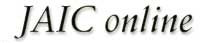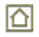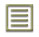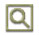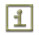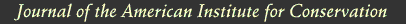JAIC 1985, Volume 24, Number 2, Article 1 (pp. 60 to 68)

### 3 REPORTING OF THE DATA

THE DATA FOR THE PEEL TESTS were calculated by averaging the results from each of the five test pulls. In the case of the MAXIMUM values, the highest peak on each run was chosen and the figure which appears in Table 1 reflects the average of those five peaks as well as the standard deviation. In the case of the MINIMUM values listed, the lowest points on each of the five runs was chosen and the figures seen reflect those averages plus or minus the standard deviation. The AVERAGE values listed were determined visually by choosing the midpoint of the graph readout.

Table 1 Summary of Data for Peel Tests of Lascaux 360 and Beva 371

The lap/shear results were obtained by recording the peaks on each of the five runs and then averaging them together. The standard deviations were also calculated.

In the discussion which follows, the AVERAGE values are used as comparisons in interpreting the results. Table 1 is printed to present the standard deviations for those that are interested.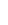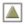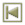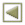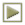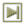Copyright � 1985 American Institute of Historic and Artistic Works